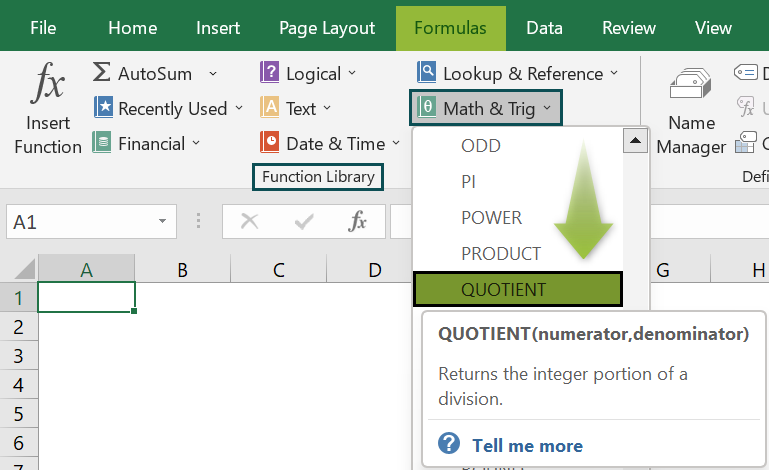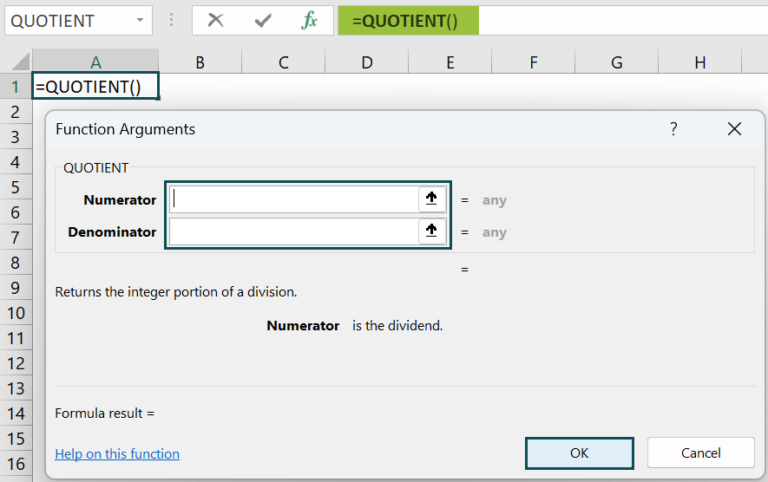# Quotient in ExcelArticle byExcelMojo Team## What Is Quotient In Excel?

The Quotient in Excel is a mathematical formulation that returns the integer value. The Quotient is the value returned on the division of the numerator and denominator.

The Quotient in Excel is categorized under the Math and Trig functions of the functional library.

For example, the image below depicts the values in columns A and B. Now, we will apply the QUOTIENT Excel Function to calculate the Quotient values as the output.

First, we should enter the Formula of the QUOTIENT Excel Function in cell B2.

So, the formula is =QUOTIENT(A2,B2).

Next, press Enter key.

The result is returned as 2.

Similarly, we can use quotient in excel function to obtain desired cells.

###### Key Takeaways
• The Quotient function in excel calculates the result by dividing the numerator by the denominator.
• It is one of the built-in functions in Excel.
• The formula of the Quotient Function is =Quotient(numerator, denominator), where the numerator is the dividend of the integer value, and the denominator is the divisor of the integer value.
• Unlike other functions, both arguments are mandatory in Quotient in Excel formula.
• We can return some messages in the place of error by using the IFERROR function in Excel.

### Syntax of Quotient() Excel Formula

• numerator: It is a dividend with an integer value.
• denominator: It is a divisor with an integer value.

Unlike other functions, all the arguments are mandatory.

### How To Use Quotient Excel Function?

• First, choose the empty cell which will contain the result.
• Go to the Formulas tab and click it.
• Next, select the Math & Trig option from the menu.
• Then, select the QUOTIENT option from the drop-down menu.
• A window called Function Arguments appears.
• As the number of arguments, enter the value in the numerator & denominator.
• Now, select OK.

2. Enter The Worksheet Manually

1. To begin with, select an empty cell for the output.
2. Next, type =QUOTIENT( in the selected cell. Alternatively, type =Q and double-click. Then, the QUOTIENT function from the list of suggestions will be shown by Excel.
3. Finally, press Enter key.

The succeeding example depicts the values, and we will calculate the Quotient value of the given values using the QUOTIENT Excel Function.

In the table,

• Column A contains the Value 1.
• Column B contains Value 2.

The steps to calculate the value by the QUOTIENT Excel Function are as follows:

1. To begin with, select the cell where we will enter the formula and then, calculate the result. The selected cell, in this case, is cell C2.

2. Next, we will enter the Formula of the QUOTIENT Excel Function in cell C2.

3. Then, enter the value of ‘numerator’ as A2, i.e., the value of the dividend.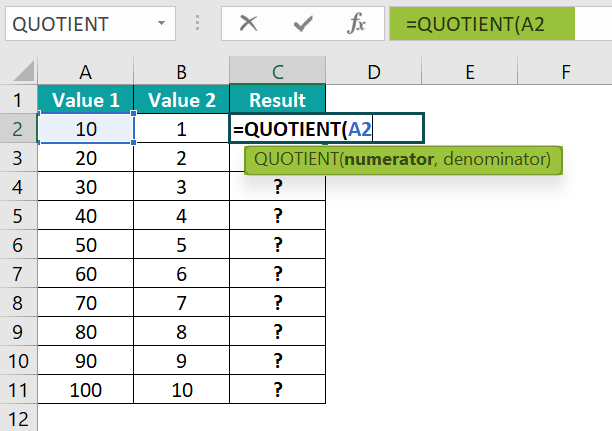4. Now, enter the value of the ‘denominator’ as B2, i.e., the value of the divisor.

5. The entered complete formula is =QUOTIENT(A2,B2) in cell C2.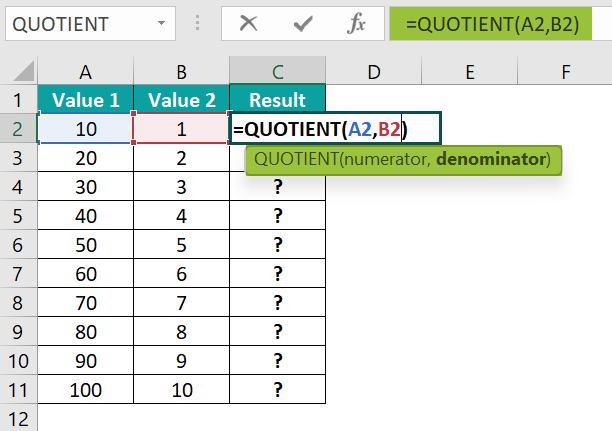6. Next, press the Enter key. The results in cell C2 are “10” in the image below.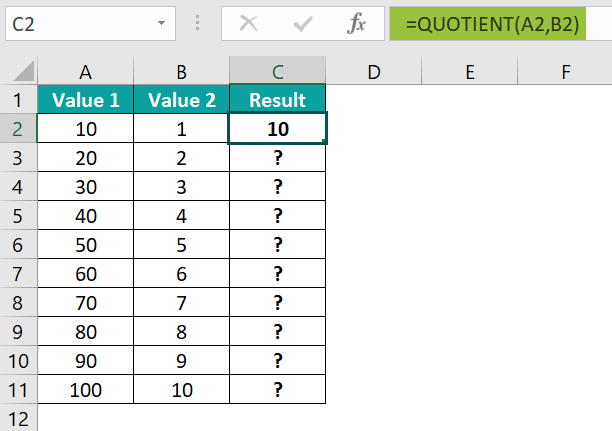7. Simialarly, press Enter and drag the cursor to cell C11, as shown in the following image.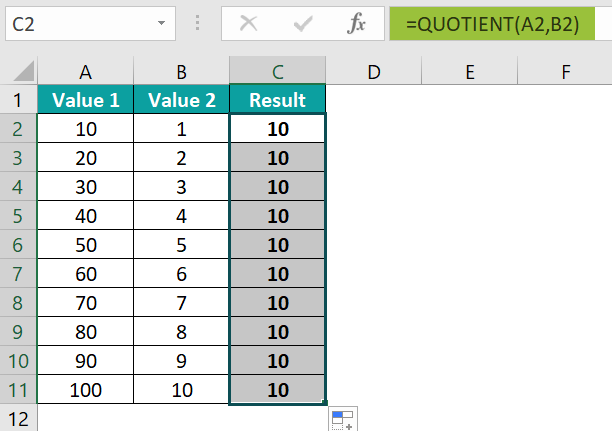Likewise, we can find quotient in excel.

### Examples

#### Example #1

The succeeding example depicts the values, and we will calculate the Quotient value of the given values using QUOTIENT Excel Function.

In the table,

• Column A shows the Value 1.
• Column B contains Value 2.

The steps to calculate the value by the QUOTIENT Excel Function are as follows:

• Step 1: First, select the cell where we will enter the formula and then, calculate the result. The selected cell, in this case, is cell C2.
• Step 2:  Next, we will enter the Formula of the QUOTIENT Excel Function in cell C2.
• Step 3: Then, enter the value of ‘numerator’ as A2, i.e., the value of the dividend.
• Step 4: Next, enter the value of the ‘denominator’ as B2, i.e., the value of the divisor.
• Step 5: The entered complete formula is =QUOTIENT(A2,B2) in cell C2.
• Step 6: Next, press the Enter key. The result in cell C2 is returned as10.
• Step 7: Now, press Enter and drag the cursor to cell C11, as shown in the following image.

Similarly, we can find Quotient in excel.

#### Example #2

The succeeding example depicts the values. Now, we will calculate the Quotient value of the given values using the QUOTIENT Excel Function and the Dividing Operator “/” and then compare the results.

In the table,

• Column A contains the Value 1.
• Column B contains Value 2.
• Column C contains the Quotient Result.
• Column D contains the Dividing Operator “/” Result.

The steps to calculate the value by the QUOTIENT Excel Function are as follows:

• Step 1: To begin with, select the cell where we will enter the formula and then, calculate the result. The selected cell, in this case, is cell C2.
• Step 2:  Next, we will enter the Formula of the QUOTIENT Excel Function in cell C2.
• Step 3: Then, enter the value of ‘numerator’ as A2, i.e., the value of the dividend.
• Step 4: Now, enter the value of the ‘denominator’ as B2, i.e., the value of the divisor.
• Step 5: The entered complete formula is =QUOTIENT(A2,B2) in cell C2.
• Step 6: Next, press the Enter key. We can see the result in cell C2 as 107.
• Step 7: Likewise, press Enter and then, drag the cursor to cell C11, as shown in the following image.
• Step 8:  Next, we will enter the Formula of the Dividing Operator “/” in cell D2.
• Step 9: So, the entered complete formula is = A2/B2 in cell D2.
• Step 10: After entering each value in the preceding step, press the Enter key. The results in cell D2 is obtained as 107.25.
• Step 11: Next, press Enter and drag the cursor to cell C11, as shown in the following image.
• Step 12: The Quotient function returns the whole number result, but the Dividing Operator “/” returns the decimal number result.

Likewise, we can find quotient in excel.

#### Example #3

The succeeding example depicts the values, and we will calculate the errors of the given values using QUOTIENT Excel Function.

In the table,

• Column A contains the Value 1.
• Column B contains Value 2.

The steps to calculate the value by the QUOTIENT Excel Function are as follows:

• Step 1: To start with, select the cell where we will enter the formula and then, calculate the result. The selected cell, in this case, is cell C2.
• Step 2: Next, we will enter the Formula of the QUOTIENT Excel Function in cell C2.
• Step 3: Then, enter the value of ‘numerator’ as A2, i.e., the value of the dividend.
• Step 4: Now, enter the value of the ‘denominator’ as B2, i.e., the value of the divisor.
• Step 5: The entered complete formula is =QUOTIENT(A2,B2) in cell C2.
• Step 6: After entering each value in the preceding step, press the Enter key. The results in cell C2  #DIV/0! error.
• Step 7: Next, press Enter and then, drag the cursor to cell C3. Now, we can see the function has returned #VALUE! error as shown below:
• Step 8: The #DIV/0! error occurs when the number is divided by 0, and the #VALUE! error occurs when the number is divided by a non-numeric value.

Likewise, we can use quotient in excel function to obtain desired cells.

### Important Things To Note

• The #DIV/0! error occurs if the supplied denominator value is blank or ‘0.’
• Remember, Quotient in Excel uses only integer values.
• #VALUE! error occurs if the supplied value is not an integer value.
• The result will be ‘0’ if the numerator is less than the denominator.
• We can use the “/” division operator if we want a decimal value, such as; “(Numerator)/(Denominator).”
• For Calculating the remainder using the same dividend and divisor, we must use the MOD function in Excel.

1. What does the QUOTIENT function do in Excel?`

The Quotient in Excel is a mathematical calculation that evaluates the result by dividing one number by another.

The syntax of the QUOTIENT function is as follows;
=QUOTIENT(numerator,denominator)

2. How does the QUOTIENT function work?

The Excel QUOTIENT Function works in Excel by following the below steps;
1) First, select an empty cell for the output.
2) Next, type =QUOTIENT( in the selected cell. Alternatively, type =Q and double-click and then the QUOTIENT function from the list of suggestions shown by Excel.
3) Then, press the Enter key.

For example, the image depicts the values, and we will calculate the Quotient value of the given values using the QUOTIENT Excel Function.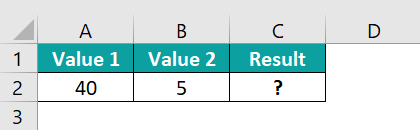The steps to calculate the value by the QUOTIENT Excel Function are as follows:

• Step 1: First, select the cell where we will enter the formula and then, calculate the result. The selected cell, in this case, is cell C2.

• Step 2:  Next, we will enter the Formula of the QUOTIENT Excel Function in cell C2.

• Step 3: Now, enter the value of ‘numerator’ as A2, i.e., the value of the dividend.

• Step 4: Enter the value of the ‘denominator’ as B2, i.e., the value of the divisor.

• Step 5: The entered complete formula is =QUOTIENT(A2,B2) in cell C2.

• Step 6: After entering each value in the preceding step, press the Enter key. The results in cell C2 is returned as 8.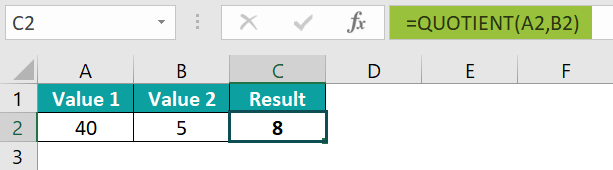Likewise, we can use quotient in excel function to obtain desired cells.

3. Where is the QUOTIENT Function in Excel?

One can activate the QUOTIENT Function in Excel using the following steps:

1) Choose the empty cell which will contain the result.
2) Go to the Formulas tab and click it.
3) Select Math & Trig from the menu.
4) Select QUOTIENT from the drop-down menu.
5) A window called Function Arguments appears.
6) As the number of arguments, enter the value in the numerator & denominator.
7) Select OK.